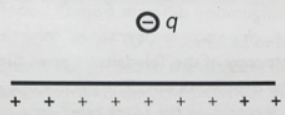# Problem: A negative charge (q = -0.75 coulombs) is placed above a floor that has a net positive charge. The floor creates a uniform electric field that has a magnitude of 105 N/C. a) What is the magnitude and direction of the electric force caused by the floor on the negative charge?  b) If the charge is initially at a distance 2.5 m above the plane, what will the change in electric potential energy (in joules) be if it is then moved to a distance 1.5 m above the plane (specify whether this change is positive/negative/zero)?

###### FREE Expert Solution

(a) Electric force:

$\overline{){\mathbf{F}}{\mathbf{=}}{\mathbf{q}}{\mathbf{E}}}$

83% (198 ratings)###### Problem Details

A negative charge (q = -0.75 coulombs) is placed above a floor that has a net positive charge. The floor creates a uniform electric field that has a magnitude of 105 N/C.a) What is the magnitude and direction of the electric force caused by the floor on the negative charge?

b) If the charge is initially at a distance 2.5 m above the plane, what will the change in electric potential energy (in joules) be if it is then moved to a distance 1.5 m above the plane (specify whether this change is positive/negative/zero)?